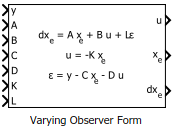Documentation

# Varying Observer Form

Observer-form state-space model with varying matrix values

• Library:
• Control System Toolbox / Linear Parameter Varying## Description

Use this block to implement a continuous-time varying state-space model in observer form. The system matrices A, B, C, and D describe the plant dynamics, and the matrices K and L specify the state-feedback and state-observer gains, respectively. Feed the instantaneous values of these matrices to the corresponding input ports. The observer form is given by:

`$\begin{array}{c}d{x}_{e}=A{x}_{e}+Bu+L\epsilon \\ u=-K{x}_{e}\\ \epsilon =y-C{x}_{e}-Du,\end{array}$`

where u is the plant input, y is the plant output, xe is the estimated state, and ε is the innovation, the difference between the predicted and measured plant output. The observer form works well for gain scheduling of state-space controllers. In particular, the state xe tracks the plant state, and all controllers are expressed with the same state coordinates.

Use this block and the other blocks in the Linear Parameter Varying library to implement common control elements with variable parameters or coefficients. For more information, see Model Gain-Scheduled Control Systems in Simulink.

## Ports

### Input

expand all

Measured plant output signal.

Plant state matrix of dimensions Nx-by-Nx, where Nx is the number of plant states.

Plant input matrix of dimensions Nx-by-Nu, where Nu is the number of plant inputs.

Plant output matrix Ny-by-Nx, where Ny is the number of plant outputs.

Plant feedforward matrix of dimensions Ny-by-Nu.

State-feedback matrix of dimensions Nu-by-Nx.

State-observer matrix of dimensions Nx-by-Ny.

### Output

expand all

Control signal (plant input).

Vector of estimated plant states.

#### Dependencies

To enable this port, select the Output states parameter.

Derivatives of the corresponding estimated states in xe.

#### Dependencies

To enable this port, select the Output state updates parameter.

## Parameters

expand all

Initial state values, specified as a scalar or a vector whose length is the number of plant states.

To identify plant states, specify state names as a:

• character vector, for a one-state plant.

• Cell array of character vectors, for a multistate plant.

Select to enable the estimated states output port, xe.

Select to enable the estimated state derivatives output port, dxe.